NEET  >  Test: Chemical Equilibrium: Heterogeneous Equilibrium

# Test: Chemical Equilibrium: Heterogeneous Equilibrium - NEET

Test Description

## 22 Questions MCQ Test Chemistry Class 11 - Test: Chemical Equilibrium: Heterogeneous Equilibrium

Test: Chemical Equilibrium: Heterogeneous Equilibrium for NEET 2023 is part of Chemistry Class 11 preparation. The Test: Chemical Equilibrium: Heterogeneous Equilibrium questions and answers have been prepared according to the NEET exam syllabus.The Test: Chemical Equilibrium: Heterogeneous Equilibrium MCQs are made for NEET 2023 Exam. Find important definitions, questions, notes, meanings, examples, exercises, MCQs and online tests for Test: Chemical Equilibrium: Heterogeneous Equilibrium below.
Solutions of Test: Chemical Equilibrium: Heterogeneous Equilibrium questions in English are available as part of our Chemistry Class 11 for NEET & Test: Chemical Equilibrium: Heterogeneous Equilibrium solutions in Hindi for Chemistry Class 11 course. Download more important topics, notes, lectures and mock test series for NEET Exam by signing up for free. Attempt Test: Chemical Equilibrium: Heterogeneous Equilibrium | 22 questions in 30 minutes | Mock test for NEET preparation | Free important questions MCQ to study Chemistry Class 11 for NEET Exam | Download free PDF with solutions
 1 Crore+ students have signed up on EduRev. Have you?
Test: Chemical Equilibrium: Heterogeneous Equilibrium - Question 1

### Direction (Q. Nos. 1-10) This section contains 10 multiple choice questions. Each question has four choices (a), (b), (c) and (d), out of which ONLY ONE option is correct. Q. Vapour pressure of NH4HS (s)is 20 mm at 25°C, for NH4HS (s)⇌ NH3(g) + NH3(g) + H2S(g) Total pressure when NH4HS (s) dissociates at 25°Cin a vessel which already contains H2S (g)at a pressure of 15 mm, is

Detailed Solution for Test: Chemical Equilibrium: Heterogeneous Equilibrium - Question 1

The correct answer is Option A.
NH4HS   ------->  NH3 + H2S
Let P be the pressure at eq. of NH3 and H2S.
Therefore, Kp = P2
= (20 / 2)2
= 100 mm
= 100
Also, Kp = (15 + P) (P)
100 = 15 P + P2
P2 + 15 P – 100 = 0
P = 5
Total pressure = 15 + 2(P)
= 15 + 2(5)
= 25 mm

Test: Chemical Equilibrium: Heterogeneous Equilibrium - Question 2

### Once the equilibrium is reached under given condition:

Detailed Solution for Test: Chemical Equilibrium: Heterogeneous Equilibrium - Question 2

In a chemical reaction, chemical equilibrium is the state in which the forward reaction rate and the reverse reaction rate are equal. The result of this equilibrium is that the concentrations of the reactants and the products do not change. However, just because concentrations aren’t changing does not mean that all chemical reaction has ceased. Just the opposite is true; chemical equilibrium is a dynamic state in which reactants are being converted into products at all times, but at the exact rate that products are being converted back into reactants. The result of such a situation is analogous to a bridge between two cities, where the rate of cars going over the bridge in each direction is exactly equal. The result is that the net number of cars on either side of the bridge does not change.

Test: Chemical Equilibrium: Heterogeneous Equilibrium - Question 3

### Ca(HCO3)2 is strongly heated and after equilibrium is attained, temperature changed to 25° C. Ca(HCO3)2(s)⇌CaO(s) + 2CO2 (g) + H2O(g) Kp = 36 (pressure taken in atm) Thus, pressure set up due to CO2 is

Detailed Solution for Test: Chemical Equilibrium: Heterogeneous Equilibrium - Question 3

The reaction is as follow:-
Ca(HCO3)2(s)⇌CaO(s) + 2CO2 (g) + H2O(g)
At 25° C H2O goes in liquid state
Kp = (PCaO)1×(PCO2)2
(PCa(HCO3)2)
Since, Ca(HCO3)2, CaO and H2O are not in gaseous state, so their partial pressure is taken 1.
Putting all values, we have
36 = (PCO2)2
Or PCO2 = 6 atm

Test: Chemical Equilibrium: Heterogeneous Equilibrium - Question 4

Na2SO4.10H2O dehydrates according to the equation,

Na2SO4· 10H2O (s)⇌ Na2sO4(s) + 10 H2O(g)

; Kp = 2.56 x 10-20

What is the pressure of water vapor at equilibrium with a mixture of Na2SO4· 10H2O and Na2sO4

Test: Chemical Equilibrium: Heterogeneous Equilibrium - Question 5

Ca(HCO3)2 decomposes as,

Ca (HCO3)2(s) ⇌ CaCO3(s) + H2O(g) + CO2(g)
Equilibrium pressure is found to be 0.12 bar. What is pco2 if the reaction mixture also contains H2O(g)at 0.20 bar?

Detailed Solution for Test: Chemical Equilibrium: Heterogeneous Equilibrium - Question 5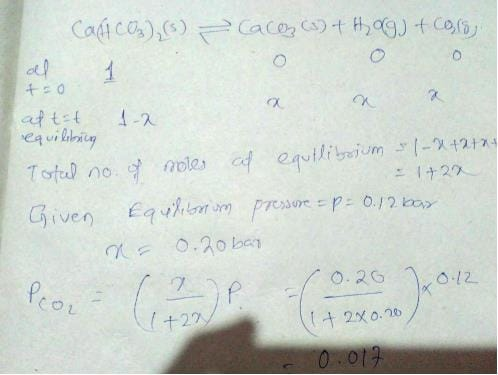Test: Chemical Equilibrium: Heterogeneous Equilibrium - Question 6

For the following equilibrium,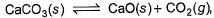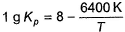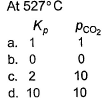Test: Chemical Equilibrium: Heterogeneous Equilibrium - Question 7

Assume following equilibria when total pressure set up in each are equal to 1 atm, and equilibrium constant (Kp) as K1; K2 and K3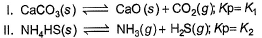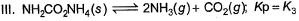Thus,

Detailed Solution for Test: Chemical Equilibrium: Heterogeneous Equilibrium - Question 7

The correct answer is option C
CaCO3 ​→ CaO + CO2​
Kp​ = k1 ​= Pco2​​
total pressure of container P
k1​ = p
NH4​HS → NH3 ​+ H2​S
PNH3​​ = PH2​S ​= P0​
P0​ + P0​ = p (total pressure)
P0 ​= p/2
k2​ = kp ​= [PNH3​​][PH2​s​] p24
NH2​CoNH2 ​→ 2NH3 ​+ CO2​
PNH3​​ = 2P0​        PCO2​ ​= P0​
2P0​ + P0 ​= P

Test: Chemical Equilibrium: Heterogeneous Equilibrium - Question 8

For the equilibrium,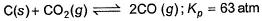at 1000 K. If at equilibrium pCO = 10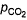then total pressure at equilibrium is

Detailed Solution for Test: Chemical Equilibrium: Heterogeneous Equilibrium - Question 8

C(s) + CO2(g) <=========> 2CO(g)
Kp = pCO2/pCO2
GIven Kp = 63 and pCO = 10pCO2
Putting the value of pCO in above equation,
63 = 100(pCO2)2/pCO2
Or pCO2 = 0.63
pCO = 6.3
Therefore, total pressure = 6.3+0.63 = 6.93 atm

Test: Chemical Equilibrium: Heterogeneous Equilibrium - Question 9

Ammonium carbamate dissociates as,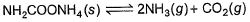In a closed vessel containing ammonium carbamate in equilibrium with its vapour, ammonia is added such that partial pressure of NH3 now equals the original total pressure. Thus, ratio of the total pressure to the original pressure is

Detailed Solution for Test: Chemical Equilibrium: Heterogeneous Equilibrium - Question 9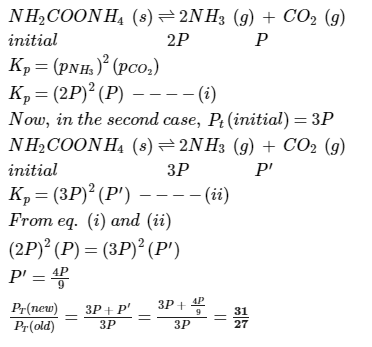Test: Chemical Equilibrium: Heterogeneous Equilibrium - Question 10

Kc forthe decomposition of NH4HS(s) is 1.8x 10-4 at 25°C.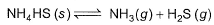If the system already contains [NH3] = 0.020 M, then when equilibrium is reached, molar concentration are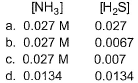Detailed Solution for Test: Chemical Equilibrium: Heterogeneous Equilibrium - Question 10

NH4HS (s)  ⇋ NH3 (g) + H2S (g)
Initial    1                   -               -
At eqm     1-x                 x+0.02     x
Kc = [NH3][H2S]   (Since NH4HS is solid, we ignore it.)
1.8×10-4    = (x+0.02)(x)
x2+0.02x-1.8×10-4 = 0
Applying quadratic formula; x = -0.02+√{(0.02)2-4×1.8×10-4}
= 0.033-0.020/2 = 0.0065
Therefore, concn of NH3 at equilibrium = x+0.020 = 0.0265
concn of H2S at equilibrium = x = 0.0065
So, option b is correct

Test: Chemical Equilibrium: Heterogeneous Equilibrium - Question 11

Direction (Q. Nos. 11-14) This section contains  a paragraph, wach describing theory, experiments, data etc. three Questions related to paragraph have been given.Each question have only one correct answer among the four given options (a),(b),(c),(d)

Passage I

Solid ammonium chloride is in equilibrium with ammonia and hydrogen chloride gases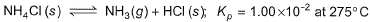0.980 g of solid NH4CI is taken in a closed vessel of 1 L capacity and heated to 275° C.

Q. Partial pressure of NH3(g) or HCI (g) at equilibrium is

Detailed Solution for Test: Chemical Equilibrium: Heterogeneous Equilibrium - Question 11

The reaction is as follow:-
NH4Cl(s)  ⇋  NH3(g) + HCl(g)
Kp = (pNH3)(pHCl)
1×10-2 = p2   (SInce reactant dissociates into same ratio, so the partial pressure will be same for both)
100×10-4 = p2
Or p = 10×10-2 = 0.10
So,  the partial pressure of NH3 and HCl are 0.10 atm.

Test: Chemical Equilibrium: Heterogeneous Equilibrium - Question 12

Passage I

Solid ammonium chloride is in equilibrium with ammonia and hydrogen chloride gases0.980 g of solid NH4CI is taken in a closed vessel of 1 L capacity and heated to 275° C.

Q. Percentage decomposition of the original sample is

Detailed Solution for Test: Chemical Equilibrium: Heterogeneous Equilibrium - Question 12

The state of HCl is given wrong. It will be in gaseous state.
So, the reaction be like;-
NH4Cl(s)  ⇌  NH3(g) + HCl(g)        kp = 1.00×10-2 at 275° C
Kp = kc(RT)2
1.00×10-2 = kc(0.0821×548)2
Or kc = 4.94×10-6
NH4Cl(s)  ⇌  NH3(g) + HCl(g)
Initial  1                     -             -
At eqm 1-x                  x            x
Kc = x2
x = √(4.94×10-6)
=  2.22×10-3
Therefore, NH4Cl dissociated at eqm = 2.22×10-3 × 53.5 = 0.118
%age decomposition = 0.118/0.980×100 = 12.13%

Test: Chemical Equilibrium: Heterogeneous Equilibrium - Question 13

Passage lI

One of the reactions that takes place in producing steel from iron ore is the reduction of iron (II) oxide by carbon monoxide to give iron metal and carbon dioxide.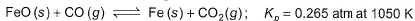Initial partial pressure
CO(g) = 1.40 atm
CO2(g) = 0.80 atm

Q. Under the given partial pressure, reaction is

Detailed Solution for Test: Chemical Equilibrium: Heterogeneous Equilibrium - Question 13

We know that with increase in pressure on one side, reaction shifts to that side which has less no. of moles(of gaseous species). However the no. of moles are same on both sides. So the REACTION WILL REMAIN IN EQUILIBRIUM.

Test: Chemical Equilibrium: Heterogeneous Equilibrium - Question 14

Passage lI

One of the reactions that takes place in producing steel from iron ore is the reduction of iron (II) oxide by carbon monoxide to give iron metal and carbon dioxide.Initial partial pressure
CO(g) = 1.40 atm
CO2(g) = 0.80 atm

Q. When equilibrium is attained,

Detailed Solution for Test: Chemical Equilibrium: Heterogeneous Equilibrium - Question 14

Initially                1.4 atm                0.80 atm
Qp = pCO2/pCO 0.8/1.4 = 0.571
SInce Qp>Kp ; reaction proceeds in the backward direction. So pressure of CO2 decreases and that of CO increases.
At eqm.               1.4+p atm            0.80-p atm
Kp = pCO2/pCO = 0.80-p/1.4+p
0.265 = 0.80-p/1.4+p
Or p = 0.339 atm
Therefore, partial pressure at eqm, pCO2 = 0.80-0.339 = 0.461 atm
And pCO = 1.4+0.339 = 1.739 atm

*Answer can only contain numeric values
Test: Chemical Equilibrium: Heterogeneous Equilibrium - Question 15

Direction (Q. Nos. 15 and 16) This section contains 2 questions. when worked out will result in an integer from 0 to 9 (both inclusive)

Hot copper tunnings can be used as an “oxygen getter" for inert gas supplies by slowly passing the gas over the copper tunnings at 650 K.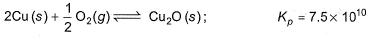Q. How many molecules of O2 are left in 1 L of a gas supply after equilibrium has been reached?

*Answer can only contain numeric values
Test: Chemical Equilibrium: Heterogeneous Equilibrium - Question 16

At 1000 K, pressure of CO2 in equilibrium with CaCO3 and CaO is equal to 2.105 atm. The equilibrium constant for the reaction,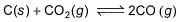is 1.9 at the same temperature when pressure are in atm. Solid C, CaO, and CaCO3 are mixed and allowed to come to equilibrium at 1000 K in a closed vessel.

Q. What is the pressure of CO (g)at equilibrium (in atm)?

Detailed Solution for Test: Chemical Equilibrium: Heterogeneous Equilibrium - Question 16

K= (partial pressure of co2/(partial pressure of co2)
since k =1.9
So 1.9 = (partial pressure of co)2/2.105
(partial pressure of co)=2.105×1.9
= 3.99 = 4
(partial pressure of co) =2

Test: Chemical Equilibrium: Heterogeneous Equilibrium - Question 17

Direction (Q. Nos. 17) Choice the correct combination of elements and column I and coloumn II  are given as option (a), (b), (c) and (d), out of which ONE option is correct.

Q. Given at 298 K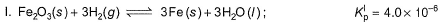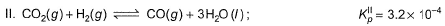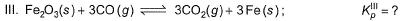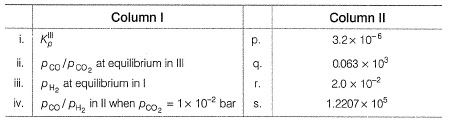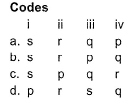Test: Chemical Equilibrium: Heterogeneous Equilibrium - Question 18

Solid ammonium carbonate (NH2CO2NH4) dissociates completely into ammonia and carbon dioxide when it evaporates :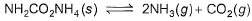At 298 K, the total pressure of the gases in equilibrium with the solid is 0.116 atm. Derive the value of equilibrium constant Kp.

Test: Chemical Equilibrium: Heterogeneous Equilibrium - Question 19

At 90° C , the following equilibrium is established :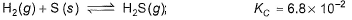If 0.20 mole of hydrogen and 1.0 mole of sulphur are heated to 90°C in a 1.0 dm3 flask, what will be the partial pressure of H2S gas at equilibrium?

Detailed Solution for Test: Chemical Equilibrium: Heterogeneous Equilibrium - Question 19

Initial moles of H₂ = 0.2
Initial moles of S = 1
Kp = 6.8 * 10⁻²
Given equation:
H2(g) + S(s) ⇋ H2S(g)
Initial moles:          0.2        1
At equilibrium: (0.2-α)   (1-α)       α
Here, in the above equation we can see that hydrogen is the limiting reagent.
∴ Kp = α/(0.2 – α)
⇒ 6.8 * 10⁻²  = α/(0.2 – α)
⇒ 1.36*10⁻² – (6.8*10⁻²)α = α
⇒ α + 0.068α = 1.36*10⁻²
⇒ α = 1.36*10⁻² / 1.068 = 1.273 * 10⁻² ← moles of H₂S
So, at equilibrium moles of H₂ = 0.2 – α = 0.2 – 1.273 * 10⁻² = 0.1873
Now, using the Ideal Gas equation,
PV = nRT ….. (i)
Where P = total pressure of the vessel
n = total no. of moles = (0.2-α) + (1-α) + α = 1.2 – α = 1.2 – 1.273*10⁻² = 1.1873
V = volume of vessel = 1 litre
R = Ideal gas constant = 0.082 L atm K⁻¹mol⁻¹
T = total temperature = 90℃ = 90+273 = 363 K
Substituting all the values in eq. (i), we get
P * 1 =  1.1873 * 0.082 * 363
⇒ P = 35.34 atm
Thus,
The partial pressure of H₂S at equilibrium
= (mole fraction of H₂S) * (total pressure)
= [1.273*10⁻² /  1.1873] * 35.34
= 0.3789 atm
≈ 0.38 atm

Test: Chemical Equilibrium: Heterogeneous Equilibrium - Question 20

Graphite is added to a vessel that contains CO2(g) at a pressure of 0.830 atm at a certain high temperature. The pressure rises due to a reaction that produces CO (g). The total pressure reaches an equilibrium value of 1.366 atm. Calculate the equilibrium constant of the following reaction.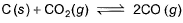Detailed Solution for Test: Chemical Equilibrium: Heterogeneous Equilibrium - Question 20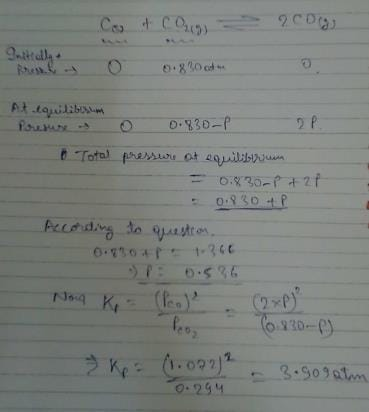Test: Chemical Equilibrium: Heterogeneous Equilibrium - Question 21

For the equilibrium,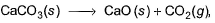Kp = 0.166 at 1000 K. Exactly 10.0 g of CaCO3 is placed in a 10.0 L flask at 1000 K. After equilibrium is reached, what mass of CaCO3 remains?

Detailed Solution for Test: Chemical Equilibrium: Heterogeneous Equilibrium - Question 21Test: Chemical Equilibrium: Heterogeneous Equilibrium - Question 22

Equilibrium constant for the reaction PCL5⇋PCL3+CL2 is 0.0205 at 230°C and 1 atmospheric pressure if at equilibrium concentration of PCL5 is 0.235 moles liter−1liter-1and that of CL2= 0.028 moleslit−1lit-1 then conc. of PCL3 at equilibrium is

Detailed Solution for Test: Chemical Equilibrium: Heterogeneous Equilibrium - Question 22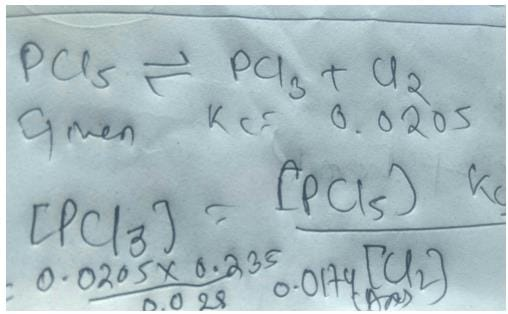## Chemistry Class 11

195 videos|334 docs|190 tests
Information about Test: Chemical Equilibrium: Heterogeneous Equilibrium Page
In this test you can find the Exam questions for Test: Chemical Equilibrium: Heterogeneous Equilibrium solved & explained in the simplest way possible. Besides giving Questions and answers for Test: Chemical Equilibrium: Heterogeneous Equilibrium, EduRev gives you an ample number of Online tests for practice

## Chemistry Class 11

195 videos|334 docs|190 tests

### How to Prepare for NEET

Read our guide to prepare for NEET which is created by Toppers & the best Teachers